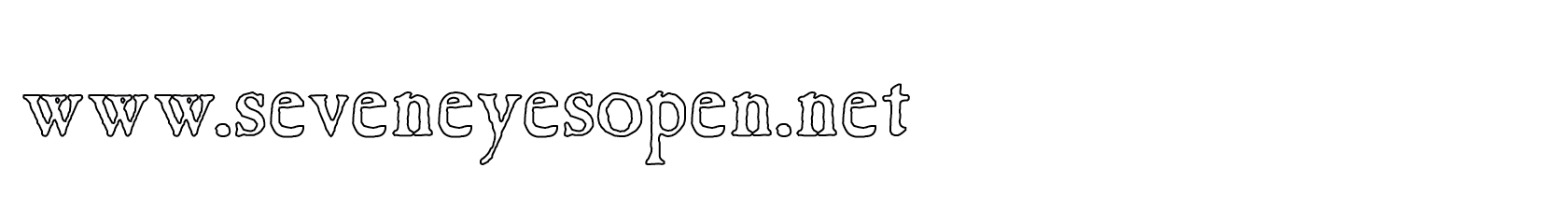None:
Polyps:
Strongs:MetaphysicsIn The Case Of Virtue I now (clearly) know that in the octal the positive property (r&s) privates a virtue in p, so therefore cannot be positive. It does not appear in the modified indexing set I under the application of those subgroups containing p from the octal itself. (Only (r&s)-1 does so.) In the case of virtue, however, (operating given virtue), I have the statement that {r} + {s} = {p, (r&s)-1} under our K4 group addition using the indexing set of the whole octal. Yet, if r&s and (r&s)-1 are not in the filter, (at all) and yet more so, not in the modified indexing set I (i.e. at all) then it can be stated that it is logically definite that {r} + {s} = {p} and {p} only. If it must logically follow that (r&s) is not positive in the group of all positive properties, then surely (r&s)-1 exists and is positive: but now given p, its negation (r&s)-1 is (crudely) not in the ultrafilter either given that particular virtue p and I may simply reduce to the product becoming {r} + {s} = {p} limiting the indexing set. Of course, given a limited indexing set, the K4 subgroup [0, p, r, s] as the set of all positive properties, the group (and the disjunction) forms an ultrafilter that does not contain r&s or (r&s)-1. Then there is no contradiction found in the equivalence of the K4 group to the ultrafilter in the three sets p, r and s at all. Why? A + B = (A v B)c leaves us with the statement that Pos((r&s)-1) must be necessarily true given p. Yet then the same statement rephrased Pos(r-1&s-1) is necessarily true and that indicates that one or both of r and/or s is not positively exemplifiable. That is it. Did you miss it? So given the virtue p, one and only one of r, s is positively exemplifiable. This is all I have to expect. (r&s)-1 leaves us with all of the information I have from the disjunction q v p&q-1. Now that q=>r and p&q-1=>s I necessitate Pos(r&s)-1 as a consequence of the freedom in the virtue p. Yet may I state that p=> (r&s)-1? If so then (r&s)-1 is a virtue! (But it cannot be so as virtue acts on one side of the disjunction q v p&q-1 only.) Truthfully I can not include (r&s)-1 as a virtue because of the group/triple {p, (r&s)-1, (u&v)} also forms a filter. The sum {r} + {s} = {p, (r&s)-1} could be reinterpreted as (r + s) = p if I can re-evaluate the argument that there are other positive properties besides r and s. (To limit the indexing set.) Clearly r&s privates p and is not positively exemplifiable despite r&s being a positive property. However I am not working in the non-principle ultrafilter of Anselm but I am here in the ultrafilter of the K4 form instead. So, r&s can never be a positive property if all that is entailed from p&q-1 is positive and truly forms an ultrafilter. (A principal one.) Now, r&s is then always "out of scope" within the K4 subgroup rather than the octal and is then not in the indexing set of all s following from p&q-1 (in the respect that q=>r), r will never appear following from p&q-1 as does s. So, given (r&s)-1 is a positive property, it must be noted it is also entailed from both sides of the disjunction! It can not be privated by either side so is always "positive" Then it is simply an observation to state that the triple {p, r, s} has no complement in the K4 ultrafilter, as the only "outside remnant" triple is (r&s)-1 which follows from the fact that at least one of r and s is positive. Then the intersection r&s is almost non-empty, given that T=(r&s)-1 follows from r and s. In fact, T entails only at most one of r and s as positive. T entails the disjunction (r v s). Given p, the same disjunction necessitates that (r&s)-1 is also a positive property. (I have an "if and only if" statement.) Then, (r&s)-1 follows from either side of the disjunction and is absent from the K4-filter given p because only one of r and s can ever be positive! So the indexing set I of the ultrafilter U contains p, r and s but not r&s or T=(r&s)-1. Each K4 group has a unique solution if it contains virtue. It is logically true that given p and r, the outcome of p+r = s. (And only s.) Likewise similarly for the other groups including one that solves p+(r&s)-1 = (u&v). All this from a simple statement that r&s is a logically impossible act to exemplify given the free exercise between r and s (given a virtue p)! After all that, the only stipulation I needed to make, was that given p, the conjunction (r&s) privates virtue in p: So that the indexing set is restricted to not include (r&s) or (r&s)-1 at all. (r&s can therefore never be positive, unless it becomes "as q".)Continue To Next Page Return To Section Start Return To Previous Page'